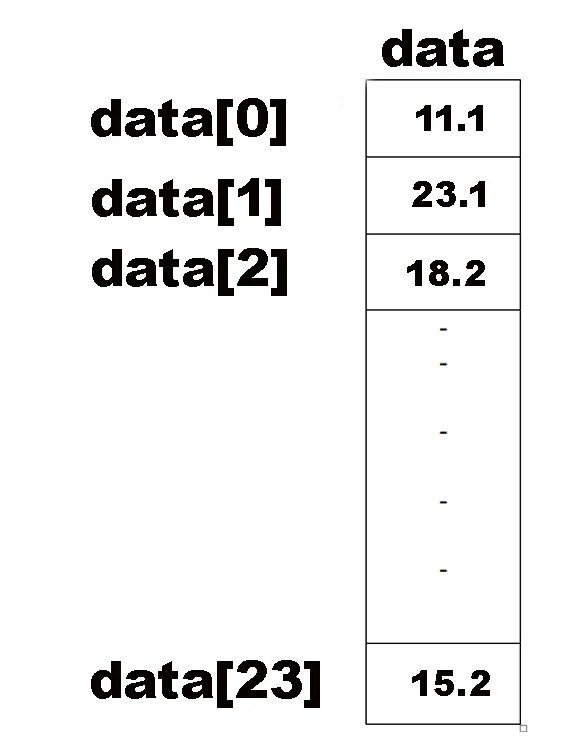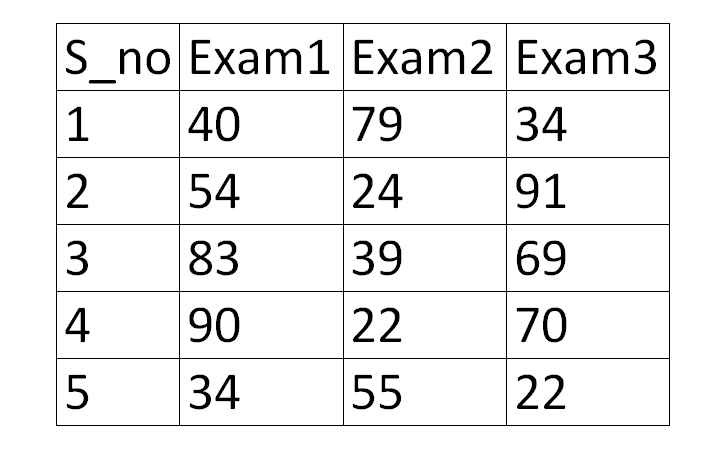# C++ array one dimensional and multidimensional with examples

(Last Updated On: March 27, 2020)## C++ Array Introduction:

C++ Array- In this tutorial, you will learn what are one dimensional and multidimensional arrays and how they are used in C++. In this tutorial, I will explain these arrays with the help of examples. After reading this article you will also be able to use arrays in Arduino programming.

Without any further delay, let’s get started!!!

## What is C++ ARRAY

A C++ Array is a powerful data structure for storing and manipulating large block of data. It is a group of related memory locations. These locations are arranged in sequence and referred to by a single name. So in this way, we can avoid having to make names for numerous items. The values in an array can either be character or numerical quantities. However, the values in an array must all be the same type i.e. all numeric or all characters.

To refer to a particular location or element within the array, we specify the name of the array and the position of the particular element within the array. For example: A, Z, N etc.

An element of an array is accessed by its subscript value. The subscript or index value is written inside a pair of square brackets[] with the name of the array. An element of the array is referenced by specifying the name of the array and the index value of the element.

Arrays are used to process a large amount of data of the same type. The data is stored in an array. The array is accessed by a single variable name. The index values are used to access individual elements of the array. A few statements are required to process data in an array. Therefore, the use of arrays in a program reduces size of the program. Arrays are divided into two types. These are:

One-dimensional arrays.
Multi-dimensional arrays.

## One dimensional C++ array:

One dimensional array is also known as a list or a linear array. It consists of only one column or one row.

For example the data of each employ of a day is stored in an array. The name of the array is “data” and its elements are data, data, data, data……..data.The above array data contains real type data. It has 24 elements. The first element of the array is data that is in position 0 and data is the 2nd element of array and is in position 1. Similarly 24th element is the last element and its reference is data.

### Declaring one dimensional array:

Like other variables an array is also declared. Defining the name of array its type and the total number of element of an array is called declaring of the array. When an array is declared a memory block with required number of location is reserved in the computer memory for storing the data into elements of array.

The general syntax to declare a one dimensional array is:

type array_name (n);

where

‘n’ is an unsigned integer value. It represents the total number of elements of the array.

To declare a one-dimensional array “data” of type double with 24 elements, the statement is written as:

double data ;

Similarly, to declare a one-dimensional array with array name “abc” having five elements and of integer type, the statement is written as:

int abc ;

### Accessing Data in One-Dimensional Arrays:

Each element of an array is referenced by its index. In an array of n elements, each element has an index value. The index of the first element is 0 and of the last element is n-1. The index value is written within square brackets after the array name. Thus the first element of an array data is referenced as data.Similarly, the last element of an array data of n elements is referenced as data[n-1].

### Input/output Data in One dimensional array

Data is entered in an array using the input statements like “cin” or “assignment” statements. It is entered into individual elements of the array. Separate input statement is used to enter data into each element of the array. Similarly to print the data from an array, the output statements are used. The data from each individual element of the array is accessed since similar input/output statement is used to access data in each element of the array, the statement is written once and a loop structure is used to repeat the input/output statement to access data of the elements of the array. In the following program, an assignment statement has been used to input data into elements of the array. The “cout” output statement has been used to print data of the elements of the array on the screen. The loop structure repeats the output statement for each individual element of the array by changing index values.

### Initializing One-Dimensional Arrays

Like other variables, the values in the elements of an array can also be assigned when the array is declared. The assigning of values to the elements of the array at the time of its declaration is called initializing of the array. For example, to declare an array “data” of type double with 5 elements, with value  44.3, 88.7, 22.2, 11.9 and 66.3 in elements data,  data,  data, data, data respectively, the statement is written as:

Double data (5] = {44.3, 88.7, 22.2, 11.9, 66.};

The values on the right-hand-side enclosed in curly brackets are assigned to the elements of  the array in the order in which they are written.

If the number of elements in an array is greater than the values in the list then the remaining last elements are initialized to zero.

## Multi-Dimensional Arrays

A multi-dimensional array is an array of more than one array.

For example, an array of two one-dimensional arrays is called two- dimensional array. It is also known as table or matrix. A two-dimensional array consists of columns and rows. Each element of the two-dimensional array is referenced by its index values or subscripts. The index value of a two-dimensional array consists of two subscripts. One subscript represents the row number and the second subscript represents the column number.

An array that requires two subscripts to identify a particular element is also known as the double-subscripted array. Consider the following figureThe above figure illustrates a table that has rows and columns and can best be by the use of two-dimensional array. Each row represents a different student and columns represent the score of the students in three different exams, to refer a single element in a two-dimensional array, it is necessary to refer to both row and column in which the item is located. For example, the result of the first student on the second exam appears in the first row and second column of the table. It would be specified as EXAM. Similarly, EXAM would be the third student’s first exam score. Notice that row reference is always list before the column reference.

So the table shown in the above figure is an example of two-dimensional  array, consisting of 5 rows and 3 columns, having 15 elements. In order to set up the required storage locations the following statement is used:

int EXAM;

### Declaration of Two-Dimensional

An A two-dimensional array is declared by giving two indexed values enclosed in square brackets. The first indexed value represents the total number of rows and the second represents the total number of columns. The syntax to declare a two-dimensional array is:

type array_name [r] [c];

where

type ——–represents the data type of array e.g. int, float, double, char, etc.

array name——represents the name of the two-dimensional array

r—-represents the total number of rows of table. It is an unsigned  number.

c ——represents the total number of columns of the table. it is an unsigned number.

C For example, to declare an integer type table “abc” having 12 and 3 columns, the declaration statement is written as:

int abc  ;

The total number of elements of the above table abc ” are 12×3=36

The first index value for the row of the above table ”abc” is 0 and the last value is 11. Similarly the first value for the column is 0 and the last value is 2.

In C++. variables, one-dimensional arrays and multi-dimensional arrays, all of same data type, can be declared in a single statement, e.g.

float a, b, c, x , y, ab ;

In the above declaration. ‘a’, ‘b’, and ‘y’ are simple variables, ‘x and ‘ab’ are two-dimensional arrays and ‘c’ is a one-dimensional array.

### Accessing Data in Two-Dimensional Arrays

Data is entered into individual elements of a two-dimensional array. To enter data, the element is referenced by its index or subscript value. Similarly, data is retrieved from an array from individual elements of the array.

Usually, nested loops are used to access elements of the two- dimensional array. The following program example inputs data into a table and then prints out the same data on the computer screen in tabular The table name is abc and it has two rows and 3 columns.

Nested loop has been used to enter data into the elements of the table. The upper loop has been used to change the index values of rows and the inner loop has been used to change the index values of columns.

When the upper loop is executed first time, the values in the first row and all its columns are entered and the value of “r” is incremented by 1.

In the second repetition of the upper loop, the value in the second row and all its columns are entered. This process is repeated till data in all elements of the table are entered. Similar method is used to print out data from the elements of the table.

## Initializing Tables

Assigning values to the elements of a table at the time of declaration is called initializing of the table.

The values are assigned to the element of a table at the time of its declaration in the same manner as they are assigned to the elements of a list.

For example, to assign values to a table abc that has two rows and three columns, values are assigned row-wise.

The declaration and initialization in the above table will be:

int abc   = {{22,55,2},{44,99,77}};

### Initializing Character Type Tables

The values in a table of char” type is also assigned in the same way as in int, float or double type tables. For example:

char st  =

{{‘a’, ‘x’, ‘y’},

{‘p’, ‘e’, ‘t’},

{‘p’, ‘a’, ‘k’},

{‘a’, ‘b’, ‘c’},

{‘3’, ‘l’. 16′}};

Each character is enclosed in single quotation marks.

The variable “st” can also be treated as a string array. In case it is taken as an array of string type, it has the capacity to store five strings each of 3 characters including null characters. Thus only two characters can be stored in each string and the null character is automatically inserted at the end.

To initialize the above data, the variable “st” is declared and initialized as:

char st(51  = { “abc”, “dog”, “game”, “fruit “, “666” };

Values assigned to string variables are enclosed in double quotation marks and are separated by commas. The length of the string is 4 characters.